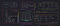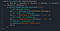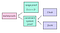# Preface

In this post, I will explain how the Bulletproofs zero knowledge proof protocol works, as well as talk about the confidential asset protocol and confidential smart contract language we are building using Bulletproofs.

This post is a condensed version of previous talks and blog posts and our Bulletproofs library documentation. It has also been featured in the MIT Digital Currency Review.

# Background

Zero-knowledge range proofs are a key building block for confidential transaction systems, such as Confidential Transactions for Bitcoin, Chain’s Confidential Assets, and many other protocols. Range proofs allow a verifier to ensure that secret values, such as asset amounts, are nonnegative. This prevents a user from forging value by secretly using a negative amount. Since every transaction involves one or more range proofs, their efficiency, both in terms of proof size and verification time, is key to transaction performance.

In 2017, Bünz, Bootle, Boneh, Poelstra, Wuille, and Maxwell published Bulletproofs, which dramatically improves proof performance both in terms of proof size and verification time. In addition, it allows for proving a much wider class of statements than just range proofs.

# Definitions

Commitment — a commitment `Com(m)` to message `m` is hiding, which means it does not reveal `m`. It is also binding, which means that if you make a commitment to `m`, you cannot open it to a different message `m’`. In the context of Bulletproofs, commitment refers to a Pedersen commitment, which has the additional property of being additively homomorphic, which means that `Com(a) + Com(b) = Com(c)` only if `a + b = c`.

Zero-knowledge proof — a proof that a certain statement is true, without revealing the secret that the statement is about. This is usually done by making a commitment to the secret that the statement is about, and sharing the commitment along with the proof.

Zero-knowledge range proof — a proof that a secret value is in a certain range (from `0` to `2^n — 1`). The proof is usually shared with a commitment to the secret value so that it can be verified.

Inner product — the sum of an entry-wise multiplication of two vectors.

Notation: vectors are written in bold, such as `a`, `2^n` (which is an `n` length vector `2^0, 2^1, ... , 2^{n-1}`), and `0^n` (which is an `n` length vector of `0`s). Inner products are written as `c = <a, b>`, where `a` and `b` are vectors of the same length, and `c` is a scalar.

# Range ProofOleg Andreev’s stylized illustration of the Bulletproofs range proof protocol

We’d like to make a proof of the following statement: `0 ≤ v < 2^n`

We know that if this is true, then `v` must be a binary number of length `n`. For example, if `n=4` and `v=3` (we’re checking if `3` is in range of `0` to `2^4 — 1`), then this means that v must be able to be broken up into a bit representation that is `4` bits long, if it is actually in range:

We would like to represent this claim in the form of an inner product because of the extremely efficient inner product proof that Bulletproof introduces, which we will talk about in the next section.

First, let’s name the bit representation of `v`: `v_bits`.

`v` should be equal to the inner product of `v_bits` and the vector `2^n` (which is an `n` length vector `2^0, 2^1, ... , 2^{n-1}`) if `v_bits` is actually the bit representation of `v`. We can do that by adding check 1 in the figure below.

Also, we need to make sure that `v_bits` is actually composed only of bits (no other pesky numbers, like `5` or `-1000`… that would be bad!) We can do that by adding check 2 in the figure below.

Next, we combine these two statements using challenge scalars, and add blinding factors to them. The math behind this is not difficult — just tedious — and ends up giving us an inner product statement in the form of `c = <a, b>`. That is, we arrive at a statement where if the statement is true, then we know that `v` is in range of `0` and `2^n`. You can follow along with the step-by-step math for how that statement is created in our range proof notes.

# Inner Product Proof

In the range proof section, I mentioned that our goal was to create a statement in the form of an inner product, for efficiency reasons. Let’s go into more detail on what an inner product is, and how we can prove an inner product efficiently!

An inner product proof is a proof that `c` is the inner product of vectors `a` and `b`. That is, `c = <a, b>`.

This may be a helpful visual for what an inner product is:

Naively, a prover could prove this to the verifier by sending over `a` and `b` and `c`, and then the verifier could verify that `c = <a, b>` by computing it themself. However, this would take `O(n)` space to send, and `O(n)` time to verify, where `n` is the length of `a` and `b`. The great thing about the bulletproofs inner product proof is that it allows us to do this proof in `O(log(n))` time and space instead!

The intuition for how the inner product proof is achieves this efficiency, is that in each step it halves the size of the `a` and `b` vectors it is working with. Therefore, it requires `log(n)` steps since after that many steps, the lengths of `a` and `b` are `1` (the base case).

So if we started with the original `a` and `b` vectors, and their product `c`, then we can divide each of the vectors into a `hi `and `lo` half:

And then, we get a random challenge scalar `x` that we use to combine the `hi `and `lo` halves of `a` and `b` to create `a’` and `b’`, which also gives us a new `c’ = <a’, b’>`.

When doing the math to expand `c’`, notice that the first two terms of `c’` are the same as `c`! Therefore, we can simplify the expression for `c’` to be written in relation to `c`, and what we can call terms `L` and `R`:

In each round, the prover sends `L` and `R` to the verifier, and repeats the next round using the `a’, b’, c’` as `a, b, c`. Notice how at each step, this halves the lengths of the vectors `a` and `b`!

After `log(n)` steps, we arrive at the base case, where `a’` and `b’` are both of length `1`. Then, there is no more compression to be done, and the prover can simply send `a’, b’, c’` to the verifier.

The verifier now has scalars `a’, b’, c’` from the base case, and scalars `L` and `R` from each of the `log(n)` steps. The verifier can then reverse the process, starting at the base case. They can trivially verify the base case by checking that `c’ = a’ * b’`. They can check that the computation is done correctly at each higher step by verifying the calculation of `c` by using the `c’, L, R` from that step, until they have completed all checks.

To see the full math behind the inner product proof, read our inner product proof notes. To see the prover and verifier’s algorithms, read our inner product proof protocol notes.

# Aggregated Range Proof

Aggregated range proofs are great for performance, because they allow `m` parties to produce an aggregated proof of their individual statements (`m` range proofs), such that the aggregated proof is smaller and faster to verify than `m` individual range proofs. The protocol for creating aggregated proofs is slightly more complex than creating individual range proofs, and requires a multi-party computation involving the parties. In our implementation, we used a centralized dealer to coordinate the communication between the `m` participating parties.

To see the math behind the aggregated proof, see our aggregated range proof notes. To see the prover and verifier’s algorithms, read our range proof protocol notes. Also of potential interest is our notes on the aggregation multi-party computation protocol and corresponding blog post.

# Constraint System Proof

A constraint system is a collection of two kinds of constraints:

Constraint systems are very powerful because they can represent any efficiently verifiable program. A zero knowledge constraint system proof is a proof that all of the constraints in a constraint system are satisfied by certain secret inputs, without revealing what those secret inputs are.

For example, we can make a set of constraints (a “gadget”) that enforces that some outputs are a valid permutation of some inputs. Let’s call this a shuffle gadgets. In a simple shuffle gadget with only two inputs and two outputs, we could represent the possible states as the following:

If we get a random scalar `x`, then we can actually express this requirement in the form of an equation: `(A — x) * (B — x) = (C — x) * (D — x)`. Because of the equality of polynomials when roots are permuted, we know that if the equation holds for a random `x`, then `{A, B}` must equal `{C, D}` in any order.

When we implement this 2-shuffle gadget in our constraint system, it looks like this:A two-shuffle gadget, which adds constraints over variables that enforce that {C, D} are a valid shuffle of {A, B}.

In line 6, we get our challenge scalar `x`.

In line 8 and 10, we make two multiplication constraints: `(A — x) * (B — x) = input_mul` and `(C — x) * (D — x) = output_mul`.

In line 12, we make one linear constraint: `input_mul — output_mul = 0`, which constrains `input_mul = output_mul`.

For the full sample code of the 2-shuffle gadget, including tests, see our GitHub repo. For an overview of how and why we implemented constraint system proofs, read our blog post here. To see the math behind constraint system proofs, read our R1CS proof notes.

# Cloak

The goal of a Confidential Assets scheme is to make transactions in which the asset value and asset type are kept hidden, thereby allowing for multi-asset transactions in which external observers cannot deduce what is being transacted but can verify that the transactions are correct. Cloak is a Confidential Assets scheme built using Bulletproofs.

Cloak is focused on one thing: proving that some number of values with different asset types is correctly transferred from inputs to outputs. Cloak ensures that values are balanced per asset type (so that one type is not transmuted to any other), that quantities do not overflow the group order (in other words, negative quantities are forbidden) and that both quantities and asset types are kept secret. Cloak does not specify how the transfers are authenticated or what kind of ledger represents those transfers: these are left to be defined in a protocol built around Cloak.

Cloak builds a constraint system using a collection of gadgets like “shuffle”, “merge”, “split” and “range proof” all combined together under a single gadget called a “cloaked transaction”. The layout of all the gadgets is determined only by the number of inputs and outputs and not affected by actual values of the assets. This way, all transactions of the same size are indistinguishable. For example, this is what a 3 input 3 output cloak transaction looks like to a verifier or a viewer of the blockchain:

This is what a 3 input 3 output cloak transaction would look like to a prover trying to make a proof of validity for a transaction with an input of \$5, ¥3, \$4 and an output of ¥3, \$6, \$3. Notice how the assets of the same type (\$) are grouped, merged together, and then split and rearranged into the target amounts:

Cloak turns out to be surprisingly efficient: compared to a single-asset transaction (such as the Confidential Transactions proposal for Bitcoin) where only range proofs are necessary, the additional constraints and gadgets needed to support the issued assets add less than 20% of the multipliers. And thanks to the inner product proof, the impact on the proof size is close to zero. This is an illustration of the number of multipliers required by each gadget in the Cloak protocol:

To learn more about Cloak, check out its specification. To see the implementation, check out the open-source Cloak GitHub repo.

# ZkVM

The goal of ZkVM is to make a smart contract language that allows for confidentiality. It builds upon previous smart contract language work, aiming for an expressive language that runs in a safe environment and outputs a deterministic log. It uses Cloak to validate that encrypted asset flows are correct, and uses Bulletproofs constraint system proofs to add constraints that values are being operated on and contracts are being executed correctly.

ZkVM is still being developed, and you can follow along with progress in the open-source ZkVM GitHub repo.

# SummaryOverview of how the pieces of this post fit together

Hopefully this post has helped you understand how the Bulletproofs zero knowledge proof protocol works, as well as what we’ve been building over that protocol. This just scratches the surface; I encourage you to read more in our notes and dive into our repo if you have more interest!

# Acknowledgements

I worked with Henry de Valence and Oleg Andreev to understand and implement the Bulletproofs paper, as well as design and implement the Cloak protocol. The protocol research team at Interstellar is currently designing and implementing ZkVM.

Thanks to Benedikt Bünz for answering all our questions about the Bulletproofs paper! Also, thanks to Andrew Poelstra for his feedback on this writeup.

Cryptographer, climber, explorer. Previously working on ZK proofs at Chain/Interstellar, now on Google’s cryptography security team.

## More from Cathie Yun

Cryptographer, climber, explorer. Previously working on ZK proofs at Chain/Interstellar, now on Google’s cryptography security team.